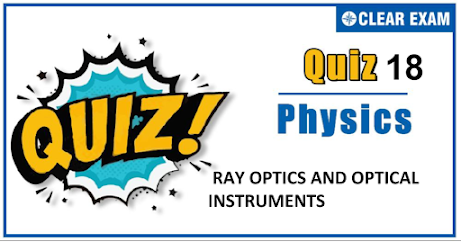## [LATEST]\$type=sticky\$show=home\$rm=0\$va=0\$count=4\$va=0

As per analysis for previous years, it has been observed that students preparing for NEET find Physics out of all the sections to be complex to handle and the majority of them are not able to comprehend the reason behind it. This problem arises especially because these aspirants appearing for the examination are more inclined to have a keen interest in Biology due to their medical background. Furthermore, sections such as Physics are dominantly based on theories, laws, numerical in comparison to a section of Biology which is more of fact-based, life sciences, and includes substantial explanations. By using the table given below, you easily and directly access to the topics and respective links of MCQs. Moreover, to make learning smooth and efficient, all the questions come with their supportive solutions to make utilization of time even more productive. Students will be covered for all their studies as the topics are available from basics to even the most advanced. .

Q1.  A thin convex lens of focal length 10 𝑐𝑚 is placed in contact with a concave lens of same material and of same focal length. The focal length of combination will be
•   Zero
•   Infinity
•   10 𝑐𝑚
•   20 𝑐𝑚
Solution
𝑓 = 𝑓1𝑓2/ 𝑓2 + 𝑓1 = 10(−10) /10 + (−10) = −100 /10 − 10 = ∞

Q2. . A thin equiconvex lens of refractive index 3/2 and radius of curvature 30 m is put in water (refractive index = 4/3 ). Its focal length is
•   0.15 m
•   0.30 m
•   0.45 m
•   1.20 m
Solution
1/𝑓 = ( (μ1/μ2) − 1)( 1/𝑅1 − 1/𝑅2 ) 1/𝑓 = ( 3/2 4/3 − 1)( 1/0.3 + 1/0.3 ) Or 1/𝑓 = ((9/8) − 1)( 2/0.3 ) Or 1/𝑓 = 1/8 × 2/0.3 or 𝑓 = 1.20 m

Q3.   An astronaut in a spaceship see the outer space as
•   White
•   Black
•   Blue
•   Red
Solution
Due to the absorption of certain wavelengths by the elements in outer layers of sun

Q4.  Critical angle is that angle of incidence in the denser medium for which the angle of reflection in rarer medium is
•
•   57°
•   90°
•   180°
Solution
90°

Q5. A ray falls on a prism 𝐴𝐵𝐶 (𝐴𝐵 = 𝐵𝐶) and travels as shown in figure. The minimum refractive of the prism material should be
•   4 3
•   √2
•   1.5
•   √3
Solution
For total internal reflection to take place 𝑖 > 𝜃𝑐 Taking sine on both sides, we get sini > sin𝜃𝑐 [as angle i at both face will be 45°] ⟹ 1/√2 > 1/𝜇 ⟹ 𝜇 > √2 .

Q6.  . In the position of minimum deviation when a ray of yellow light passes through the prism, then its angle of incidence is
•   Less than the emergent angle
•   Greater than the emergent angle
• Sum of angle incidence and emergent angle is 90°
•   Equal to the emergent angle
Solution
In minimum deviation position ∠𝑖 = ∠𝑒

Q7. To increase the magnifying power of telescope (𝑓o = focal length of the objective and 𝑓e = focal length of the eye lens)
•   𝑓0 should be large and 𝑓e should be small
•   𝑓0 should be small and 𝑓e should be large
•   𝑓o and 𝑓e both should be large
•   𝑓o and 𝑓e both should be small
Solution

By formula 𝑚 = 𝑓o/𝑓e

Q8. . A lens is placed between a source of light and a wall. It forms images of area 𝐴1 and 𝐴2 on the wall for its two different positions. The area of the source or light is
•   [𝐴1 + 𝐴2]/2
• [ 1/𝐴1 + 1/𝐴2 ]-1
•   √𝐴1𝐴2
•   [(√𝐴1 + √𝐴2)/2 ]2
Solution
𝑚1 = 𝐴1/𝑂 and 𝑚2 = 𝐴2/𝑂 ⇒ 𝑚1𝑚2 = 𝐴1𝐴2/𝑂2 Also it can be proved that 𝑚1𝑚2 = 1 So 𝑂 = √𝐴1𝐴2

Q9.An achromatic combination of lenses produces
•   Images in black and white
•   Coloured images
•   Images unaffected by variation of refractive index with wavelength
•   Highly enlarged images are formed
Solution
The image of an object in white light formed by a lens is usually coloured and blurred. This defect of image is called chromatic aberration and arises due to the fact that focal length of a lens is different for different colours. In case of two thin lenses in contact, the combination will be free from chromatic aberration. The lens combination which satisfies this condition is called achromatic lenses.

Q10. A diminished virtual image can be formed only in
•   Plane mirror
•   A concave mirror
•   A convex mirror
• Concave-parabolic mirror
Solution
A convex mirror## Want to know more

Please fill in the details below:

## Latest NEET Articles\$type=three\$c=3\$author=hide\$comment=hide\$rm=hide\$date=hide\$snippet=hide

Name

ltr
item
BEST NEET COACHING CENTER | BEST IIT JEE COACHING INSTITUTE | BEST NEET, IIT JEE COACHING INSTITUTE: ray optics and optical instruments quiz 18
ray optics and optical instruments quiz 18